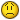Cell Range Split into Multiple Cells

W

Willie

I have a cell range (A1:A4) that contains the following information

A1
When Assigned Organization is XYZ, change Assigned POC to Black, Jack,
change Assigned Alt. POC to Doe, Jane, and change Substitute POC to
White, Jack

A2
When Assigned Department is X-A/7, change Assigned POC to Young, Jim,
change Assigned Alt. POC to Brown, Frank, and change Substitute POC to
Stewart, Rod

A3
When Assigned Group is X-B (XMO/ABC), change Assigned POC to Simon,
Paul, change Assigned Alt. POC to Bowie, David, and change Substitute
POC to Miller, Steve

A4
When Assigned Staff is Staffing Personnel (SP), change Assigned POC to
Gates, David, change Substitute POC to Reed, Lou, and change Assigned
Alt. POC to Gaines, Steve

Note that the strings above are not always in the same order. Compare
the POCs for A3 & A4

I need to split this cell range into several different columns. I
need cells B1:B4 to contain the string of characters between “When”
and “is”. For example, B1:B4 will contain the following:
Assigned Organization
Assigned Department
Assigned Group
Assigned Staff

I need cells C1:C4 to contain the string of characters between “is”
and the first “,”. C1:C4 will contain the following:
XYZ
X-A/7
X-B (XMO/ABC)
Staffing Personnel (SP)

D14 will contain the “Assigned POC”. E1:E4 will contain the
“Assigned Alt. POC” and F1:F4 will contain the “Substitute POC”
Again, The POCs are not always in the same order...

I’ve tried the RIGHT, LEFT, LEN and FIND functions but I can’t get
the
correct combination to solve this. Can anyone help?

I

isabelle

hi Willie,

=MID(A1,SEARCH("is",A1)+3,SEARCH(", change",A1)-SEARCH("is",A1)-3)

W

Willie

hi Willie,

=MID(A1,SEARCH("is",A1)+3,SEARCH(", change",A1)-SEARCH("is",A1)-3)
Isabelle,

Thanks for your help. This got me going but I still have an Error for
the POCs since they are not in a uniform order.

The first column works great using:
=MID(\$A1,SEARCH("When",\$A1)+5,SEARCH(" is",\$A1)-SEARCH("When",\$A1)-5)
And produces the following:
Assigned Organization
Assigned Department
Assigned Group
Assigned Staff

The second column works with:
=MID(\$A1,SEARCH("is",\$A1)+3,SEARCH(", change",\$A1)-SEARCH("is",\$A1)-3)
Produces:
XYZ
X-A/7
X-B (XMO/ABC)
Staffing Personnel (SP)

The POCs are causing me pain. I’m using:
=MID(\$A1,SEARCH("Assigned POC",\$A1)+15,SEARCH(", change Assigned Alt.",
\$A1)-SEARCH("Assigned POC",\$A1)-15)
Which works ok for the first three but produces an error for the 4th.
The diff POCs can be anywhere in the string...
Black, Jack
Young, Jim
Simon, Paul
#VALUE!

For “Assigned POC” I really need the 2nd string of “,change” for my
2nd Search. Or the second "," to the RIGHT of the first SEARCH for
“Assigned POC”

W

Willie

Thanks for your help. This got me going but I still have an Error for
the POCs since they are not in a uniform order.

Given that new information, I have modified my VBA Macro:

==================================
Option Explicit
Sub ParsePOC()
Dim rg AsRange, c AsRange
Dim re As Object, mc As Object
Dim s As String
Dim i As Long, j As Long
Dim sPat As Variant
Set rg =Range("A1",Cells(Rows.Count, "A").End(xlUp))
Set re = CreateObject("vbscript.regexp")
With re
.Global = True
.MultiLine = False
.ignorecase = True
End With
sPat = Array("When\s+([\s\S]+?)(?=\s+is)", _
"is\s+([^,]+)", _
"Assigned\s+POC\s+to\s+([\s\S]+?)(?=,(??:\s*and\s*change)|(?:\s*change))|­\$)", _
"Alt\.\s*POC\s*to\s*([\s\S]+?)(?=,(??:\s*and\s*change)|(?:\s*change))|\$)"­, _
"Substitute\s*POC\s*to\s*([\s\S]+?)(?=,(??:\s*and\s*change)|(?:\s*change)­)|\$)")

Range(rg(1, 2), rg(rg.Rows.Count - 1, 6)).ClearContents
For Each c In rg
re.Pattern = "[\r\n]+"
s = Trim(re.Replace(c.Text, " "))
For i = LBound(sPat) To UBound(sPat)
re.Pattern = sPat(i)
If re.test(s) = True Then
Set mc = re.Execute(s)
c(1, i - LBound(sPat) + 2).Value = mc(0).submatches(0)
End If
Next i
Next c

End Sub
========================================

Thanks Ron...this worked very nice. I move my data so I ended up with
this.

Option Explicit
Sub AssigmentParsePOC()
Dim rg As Range, c As Range
Dim re As Object, mc As Object
Dim s As String
Dim i As Long, j As Long
Dim sPat As Variant

'Where to start
'Set rg = Range("A1", Cells(Rows.Count, "A").End(xlUp))
Set rg = Range("C2", Cells(Rows.Count, "C").End(xlUp))

Set re = CreateObject("vbscript.regexp")
With re
.Global = True
.MultiLine = False
.ignorecase = True
End With

sPat = Array("When\s+([\s\S]+?)(?=\s+is)", _
"is\s+([^,]+)", _
"Assigned\s+POC\s+to\s+([\s\S]+?)(?=,(??:\s*and
\s*change)|(?:\s*change))|\$)", _
"Alt\.\s*POC\s*to\s*([\s\S]+?)(?=,(??:\s*and\s*change)|
(?:\s*change))|\$)", _
"Substitute\s*POC\s*to\s*([\s\S]+?)(?=,(??:\s*and
\s*change)|(?:\s*change))|\$)", _
"Substitute-2\s*POC\s*to\s*([\s\S]+?)(?=,(??:\s*and
\s*change)|(?:\s*change)­)|\$)")

Range(rg(1, 2), rg(rg.Rows.Count - 1, 6)).ClearContents
For Each c In rg
re.Pattern = "[\r\n]+"
s = Trim(re.Replace(c.Text, " "))
For i = LBound(sPat) To UBound(sPat)
re.Pattern = sPat(i)
If re.test(s) = True Then
Set mc = re.Execute(s)
c(1, i - LBound(sPat) + 2).Value = mc(0).submatches(0)
End If
Next i
Next c